# Test: Three Phase Converters - 1

## 20 Questions MCQ Test Power Electronics | Test: Three Phase Converters - 1

Description
Attempt Test: Three Phase Converters - 1 | 20 questions in 20 minutes | Mock test for Electrical Engineering (EE) preparation | Free important questions MCQ to study Power Electronics for Electrical Engineering (EE) Exam | Download free PDF with solutions
QUESTION: 1

### A three-phase, three-pulse, M-3 type controlled converter uses ____________ number of SCRs.

Solution:

It uses three SCRs with a three-phase transformer. M-3 type 3-pulse converters are not practically used.

QUESTION: 2

### A three-phase, three-pulse, M-3 type controlled converter has firing angle for one of the SCRs set as 15°. This SCR would start conducting at

Solution:

In a three phase controller, the actually conduction starts at 30° + α. Hence, ωt = 30+15 = 45°.

QUESTION: 3

### In a three-phase, three-pulse, M-3 type controlled converter T1 starts to conduct at 30 + n°. At what angles do T2 and T3 start to conduct? Assume that the conduction sequence is T1-T2-T3.

Solution:

In three-phase three pulse converter the conduction can start only after 30°. As each SCR conducts from 120°, T2 would conduct on 30+120+n° = 150+n° and so on.

QUESTION: 4

A three-phase three pulse type controlled converter is constructed using 3 SCR devices. The circuit is supplying an R load with α < 30°. As such, each SCR device would conduct for

Solution:

Each conduct for 120° per cycle is the firing angle is less than 30°. 120 x 3 = 360°.

QUESTION: 5

Find the expression for average output voltage at R for the below given configuration. Take firing angle as α = 15°, transformer ratio as 1:1:1 and Vmp as the maximum value of phase voltage at the supply.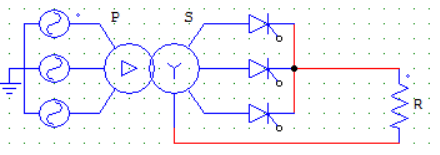Solution:

The circuit is that of a three-pulse M-3 connection. The firing angle is less than 30°. Therefore, each device conducts for an angle of 120°.
Vo = 3 x [ 1/2π ∫ Vmp sin⁡ωt d(ωt) ] Where, the integration runs from α+π/6 to α+5π/6.
Vo = (3√3/2π) x Vmp x cosα.

QUESTION: 6

In the circuit shown below, SCR T1 conducts first. If T1 is fired at an angle of α > 30°, then T1 would conduct from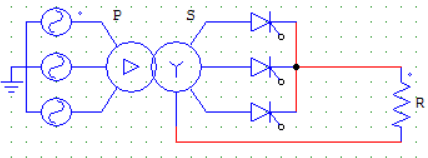Solution:

When firing angle is more than 30°, T1 would conduct from 30 + α to 180°. Irrespective of the firing angle, T1 will be turned on at 180° because it conducts first which means it is connected to the R phase and the phase sequence is R-Y-B. As R starts at 0° its value is 0 at 180° which reverse biases the SCR T1.

QUESTION: 7

Find the expression for average output voltage for the given circuit if firing angle is greater than 30°. Take Vmp = secondary side maximum value of phase voltage.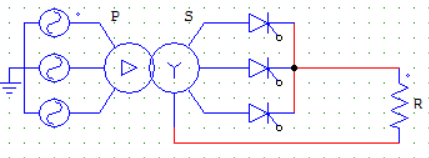Solution:

Vo = 3 x [ 1/2π ∫ Vmp sin⁡ωt d(ωt) ] Where, the integration runs from α+π/6 to π. Because conduction takes place from 30 + α to 180° for T1 and than the waveform is symmetrical for all other SCRs.
Vo = (3√3/2π) x Vmp x [1+cos(30+α)].

QUESTION: 8

A three-phase three-pulse converter would operate as a line commutated inverter when

Solution:

The output voltage is proportional to cosine of the firing angle α. If α goes above 90° then the output voltage is negative, meaning that it is operating as an inverter.

QUESTION: 9

A three-phase M-3 converter is operated from a 3-phase, 230 V, 50 Hz supply with load resistance R = 10 Ω. Find the value of firing angle if an average output voltage of 50% of the maximum possible output voltage is required.Hint: α > 30°.

Solution:

We need , Vo = 0.5 Vom.
α>30° hence we use the equation Vo = (3√3/2π) x Vmp x [1+cos(30+α)] √3Vmp = Vml = √2×230
Therefore, Vo = (3/2π) x √3 Vml x [1+cos(30+α)] = 0.5Vom
(1/√3) x [1+cos(30+α)] = Vo x 2π/3Vml = Vo/Vom = 1/2
α = 67.7°.

QUESTION: 10

A three-phase half-wave controlled converter is fed from a 3-phase, 400 V source and is connected to a load which takes a constant current of 36 A. Find,the value of average output voltage and average current rating of SCR for a firing angle of 30°.

Solution:

Vo = (3√3/2π) x Vmp x cosα = 233.874 V.
Ia = Io/3 = 12 A.

QUESTION: 11

A three phase full converter will require __________ number of SCRs.

Solution:

Three legs having two SCRs each, six in total.

QUESTION: 12

A three phase six pulse full converter works as a ac to dc converter for firing angles in the range

Solution:

When α is less than 90°, the SCRs conduct for 120° and the current and voltage are positive on an average hence, the power flows from AC source to DC load.

QUESTION: 13

For the below given circuit, α = 60°. T2 will start conduction at ωt = __________ Assume the inductor L value to be negligible.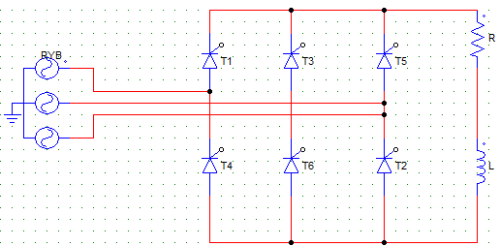Solution:

Assuming the phase sequence is R-Y-B. T1 would start conducting at 30+60 = 90°, T2 at 90+210/2 = 150°. This is because after T1, T3 would conduct from the upper group, as T2 belongs to the lower group it will start to conduct exactly between T1 and T3 i.e. between 90 and 210(90+120) which is 150°.

QUESTION: 14

For a three phase full controlled converter, with 3 thyristors in the upper or positive group and 3 thyristors in the lower or negative group, at any given time

Solution:

Let’s say T1, T3 and T5 belong to the positive group and T2, T4 and T6 to the negative group. At any given time one SCR from each group conducts. e.g. T1 and T6 or T1 and T2.

QUESTION: 15

In case of a three phase full controlled converter with 6 SCRs, commutation occurs every

Solution:

Every SCR conducts for 120°. This means that the SCRs from the positive group are fired 120° among themselves, same is true for SCRs from negative group. For example, if T1 starts conducting at 90° it will conduct till 90+120 = 210°. But while T1 is conducting, half of the time i.e. from 90 to 150, T6 is conducting and another half of the time T2 is conducting. Hence, commutation (change in the SCR which is conducting) takes place every 60 degrees irrespective of the firing angle. Construct the firing sequence table for better understanding.

QUESTION: 16

For the below given circuit, the conduction sequence for the negative group of SCRs is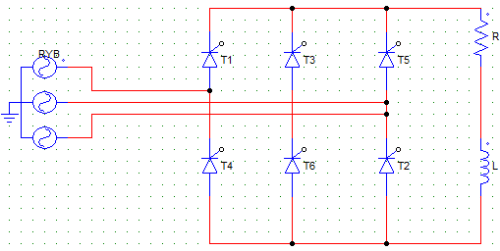Solution:

The negative group of SCRs has T2, T4 and T6. The conduct as T2-T4-T6, as T2 is connected to the B phase, T4 to the R phase and like-wise.

QUESTION: 17

For a three-phase full controlled converter with R load, the average value of output voltage is zero for

Solution:

For α = 90 degrees, the voltage waveform is equally symmetrical about the ωt axis, hence the average value is zero. This can also be found by using the formula for average output voltage,
Vo = (3Vml/π) cos α,
For α = 90°, cosα = 0, Vo = zero.

QUESTION: 18

A three-phase full converter charges a battery from a three-phase supply of 230 V. The battery emf is 200 V and the internal resistance of the battery is 0.5 Ω. Find the value of the continuous current which is flowing through the battery if its terminal voltage is 210 V

Solution:

Vo = 210 V
Vo = E + Io x R
210 = 200 + 0.5 x Io
Io (Current through the battery) = 20 A.

QUESTION: 19

A three-phase full converter charges a battery from a three-phase supply of 230 V. The battery emf is 200 V. Find the value of the firing angle if the battery terminal voltage is 210 V.

Solution:

Vo = (3Vml/π) cos α
α = cos-1(210π/3√2×230) = 47.453°.

QUESTION: 20

A three-phase full converter charges a battery from a three-phase supply of 230 V. Find the value of the power delivered to the load if a continues current of 20A is flowing through the battery of emf 200 V and internal resistance of 0.5 Ω.

Solution:

Iavg = Irms = 20 A
P = E x Iavg + Irms2 x R = 4200 W.Use Code STAYHOME200 and get INR 200 additional OFF Use Coupon Code